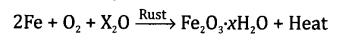# Class 10 Science Chapter 1 Chemical Reactions and Equations

NCERT Solutions Class 10 Science Chapter 1 Chemical Reactions and Equations.

Question 1.

Why should magnesium ribbon be cleaned before burning in air?
Magnesium is a reactive metal. When it is exposed to atmosphere for longer time, the oxygen present in the atmosphere combines and MgO is formed on the metal surface. This oxide layer does not burn in the flame so magnesium ribbon has to be cleaned with sandpaper before burning in air.

Question 2.
Write the balanced equations for the following chemical reactions:
(a) Hydrogen + Chlorine ➝ Hydrogen chloride
(b) Barium chloride + Aluminium sulphate ➝ Barium sulphate + Aluminium chloride
(c) Sodium + Water ➝ Sodium hydroxide + Hydrogen
The balanced equations are written as:
(a) H 2 + Cl 2 ➝ 2HCl
(b) 3BaCl 2 + Al 2(SO 4) 3 ➝ 3BaSO 4 + 2AlCl 3
(c) 2Na + 2H 2O ➝ 2NaOH + H2

Question 3.
Write the balanced equations with state symbols for the following reactions:
(a) Solutions of barium chloride and sodium sulphate in water react to give insoluble barium sulphate and the solution of sodium chloride.
(b) Sodium hydroxide solution (in water) reacts with hydrochloric acid solution (in water) to produce sodium chloride and water.
The symbol equations in balanced form for the reactions are:
(a) BaCl 2(aq) + Na 2SO 4(aq) ➝ BaS0 4(s)+ 2NaCl(aq)
(b) NaOH(aq) + HCl(aq) ➝ NaCl(aq) + H 2O(l)

Question 4.
A solution of the substance ‘X’ is used for white washing.
(a) Name the substance ‘X’ and write its formula.
(b) Write the reaction of the substance ‘X’ with water.
(a) The substance ‘X’ is calcium oxide (also called quick lime). Its formula is CaO.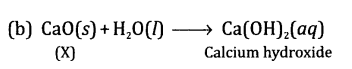Question 5.
Why is the amount of gas collected in one of the test tubes in Activity 7 double of the amount collected in the other? Name the gas.
It is so because in water, the hydrogen is two times more than oxygen by mass and volume. The equation is expressed as:Here the two gases have volume in ratio of 2 : 1. The gas is hydrogen.

Question 6.
When you mix solutions of lead(II) nitrate and potassium iodide.
(a) What is the colour of the precipitate formed? Name the compound involved.
(b) Write a balanced chemical equation for the reaction.
(c) What type of a reaction is this?
(a) The precipitate is yellow in colour. The compound is lead(II) iodide with chemical formula PbI 2.
(b) Pb(NO 3) 2(aq) + 2KI(aq) ➝ Pbl 2(s) + 2KNO 3(aq)
(c) It is a double displacement reaction.

Question 7.
Why does the colour of copper sulphate change when an iron nail is dipped in it?
Iron nail acquires a brown coating of copper as a result of displacement reaction. The solution becomes light green.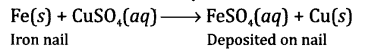Question 8.
Give one example of the double displacement reaction.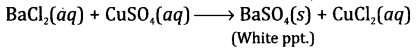Question 9.
Identify the substances that are oxidised and the substances that are reduced in the following reactions:
(a) 4Na(s) + O 2(g) ➝ 2Na 2O(s)
(b) CuO(s) + H 2(g) ➝ Cu(s) + H 2O(l)
(a) In this reaction, sodium (Na) is oxidised to sodium oxide (Na 2O). This means that oxygen (O 2) gets reduced.
(b) In this reaction, hydrogen (H 2) is oxidised to form water (H 2O) while copper(II) oxide (CuO) is reduced to copper (Cu).

Question 10.
Magnesium ribbon burns with a dazzling flame in air (or oxygen) and changes to a white substance magnesium oxide. Is magnesium being oxidised or reduced in this reaction?
The balanced chemical equation is:
2Mg(s) + O 2(g) ➝ 2MgO(s)
Yes, magnesium (Mg) gets oxidised to form magnesium oxide (MgO) in this reaction.

is same as the total mass of products. It implies mass can neither be created nor be destroyed during a chemical reaction.

Chapter End Questions

Question 1.
Which of the following statements about the reaction given below are incorrect?
2PbO(s) + C(s) ➝ 2Pb(s) + CO2(g)
(ii) Carbqn dioxide is getting oxidised.
(iii) Carbon is getting oxidised.
(iv) Lead oxide is getting reduced.
(a) (i) and (ii)
(b) (i) and (iii)
(c) (i), (ii) and (iii)
(d) all are incorrect
(a) (i) and (ii)

Question 2.
Fe 2O 3 + 2Al ➝ Al 2O 3 + 2Fe
The above reaction is an example of:
(a) combination reaction
(b) double displacement,reaction
(c) decomposition reaction
(d) displacement reaction
(d) displacement reaction

Question 3.
What happens when dilute hydrochloric acid is added to iron filings? Tick the correct answer.
(a) Hydrogen gas and iron chloride are produced.
(b) Chlorine gas and iron hydroxide are produced.
(c) No reaction takes place.
(d) Iron salt and water are produced.
(a) Hydrogen gas and iron chloride are produced.

Question 4.
What is a balanced chemical equation? Why should chemical equations be balanced? 
A chemical equation is said to be balanced if:
(a) the atoms of different elements on both sides of the equations are equal.
(b) the equation is molecular, i.e. the gases if involved in the equation must be in the molecular form (e.g. H 2, O 2, N 2, Cl 2, etc.).
Necessity to balance chemical equations: The chemical equations have to be balanced to fulfil the requirement of law of conservation of mass. According to the law, the total mass of reactants

Question 5.
Transfer the following into chemical equations and balance them:
(a) Hydrogen gas combines with nitrogen to form ammonia.
(b) Hydrogen sulphide gas burns in air to give water and sulphur dioxide.
(c) Barium chloride reacts with aluminium sulphate to give aluminium chloride and precipitate of barium sulphate.
(d) Potassium metal reacts with water to give potassium hydroxide and hydrogen gas.
(a) The symbol equation for the reaction is:
H 2 + N 2 ➝ NH 3
The balancing of equation is done in the following steps:
Step I: Let us count the number of atoms of all the elements of the reactants and the products on both sides of the equation.

 Elements No. of atoms of reactants (LHS) No. of atoms of products (RHS) H 2 3 N 2 1

Step II: In order to equate the number of H atoms on both sides, put coefficient 3 before H 2 on the reactant side and coefficient 2 before NH 3 on the product side.
3H 2 + N 2 ➝ 2NH 3
Step III: On counting, the number of N atoms on both sides of the equation are same. This means that the equation is balanced.
Step IV: The balanced equation can be written in physical state as:
3H 2(g) + N 2(g) ➝ 2NH 3(g)

(b) The symbolic equation for the reaction is:
H 2S + O 2 ➝ H 2O + SO 2
The balancing of equation is done in the following steps:
Step I: Let us count the number of atoms of all the elements on both sides of the equation.

 Elements No. of atoms of reactants (LHS) No. of atoms of products (RHS) H 2 2 S 1 1 O 2 3

Step II: In order to equate the number of O atoms, put coefficient 3 before O2 on the reactant side and coefficient 2 before SO2 on the product side.
H 2S + 3O 2 ➝ H 2O + 2SO 2
Step III: 0 atoms are still not balanced. To achieve this, put coefficient 2 before H 2O on the product side.
H 2S + 3O 2 ➝ 2H 2O + 2S0 2
Step IV: To balance S atoms, put coefficient 2 before H 2S on the reactant side.
2H 2S + 3O 2 ➝ 2H 2O + 2SO 2
Step V: On inspection, the number of atoms of all the elements on both sides of the equation are equal. Therefore, the equation is balanced.
Step VI: The balanced equation can be written in physical state as:
2H 2S(g) + 3O 2(g) ➝ 2H 2O(g) + 2SO 2(g)

(c) The symbolic equatipn for the reaction is:
BaCl 2 + Al 2(SO 4) 3 ➝ AlCl 3 + BaSO 4
The balancing of equation is done in the following steps:
Step I: Let us count the number of atoms of all the elements on both sides of the equation.

 Elements No. of atoms of reactants (LHS) No. of atoms of products (RHS) Ba 1 1 Al 2 1 Cl 2 3 S 3 1 O 12 4

Step II: In order to equate the number of Al atoms, put coefficient 2 before AlCl3 on the product side.
BaCl 2 + Al 2(SO 4) 3 ➝ 2AlCl 3 + BaSO 4
Step III: In order to balance Cl atoms, put coefficient 3 before BaCl 2 on the reactant side.
3BaCl 2 + Al 2(SO 4) 3 ➝ 2AlCl 3 + BaSO 4
Step IV: To balance Ba atoms, put coefficient 3 before BaSO 4 on the product side.
3BaCl 2 + Al 2(SO 4) 3 ➝ 2AlCl 3 +3BaSO 4
Step V: On inspection, the number of S and 0 atoms on both sides of the equation are also found to be equal. Thus, the equation is in balanced form.
Step VI: The balanced equation can be written in physical state as:
3BaCl 2(aq) + Al 2(SO 4) 3(aq) ➝ 2AlCl 3(aq) + 3BaSO 4(s)

(d) The symbolic equation for the reaction is:
K + H 2O ➝ KOH + H 2
The balancing of equation is done in the following steps:
Step I: Let us count the number of atoms of all the elements on both sides.

 Elements No. of atoms of reactants (LHS) No. of atoms of products (RHS) K 1 1 H 2 3 O 1 1

Step II: To balance the number of H atoms, put coefficient 2 before KOH on the product side and 2 before H 2O on the reactant side.
K + 2H 2O ➝ 2KOH + H 2
Step III: To balance the number of K atoms in the above equation, put coefficient 2 before K atom on the reactant side.
2K + 2H 2O ➝ 2KOH + H 2
Step IV: On inspection, the number of atoms of all the elements are found to be equal on both sides of the equation. It is finally balanced.
Step V: The balanced equation can be written in physical state as:
2K(s) + 2H 2O(l) ➝ 2KOH(aq) + H 2(g)

Question 6.
Balance the following chemical equations:
(a) HNO 3 + Ca(OH) 2 ➝ Ca(NO 3) 2 + H 2O
(b) NaOH + H 2SO 4 ➝ Na 2SO 4 + H 2O
(c) NaCl + AgNO 3 ➝ AgCl + NaNO 3
(d) BaCl 2 + H 2SO 4 ➝ BaSO 4 + HCl
(a) 2HNO 3 + Ca(OH) 2 ➝ Ca(NO 3) 2 + 2H 2O
(b) 2NaOH + H 2SO 4 ➝ Na 2SO 4 + 2H 2O
(c) The symbolic equation as given for the reaction is already balanced.
NaCl + AgNO 3 ➝ AgCl + NaNO 3
(d) BaCl 2 + H 2SO 4 ➝ BaSO 4 + 2HCl

Question 7.
Write the balanced equations for the following reactions:
(a) Calcium hydroxide + Carbon dioxide ➝ Calcium carbonate + Water
(b) Aluminium + Copper chloride ➝ Aluminium chloride + Copper
(c) Barium chloride + Potassium sulphate ➝ Barium sulphate + Potassium chloride
(d) Zinc + Silver nitrate ➝ Zinc nitrate + Silver
All these equations are in word form whereas the balanced equations are written in symbolic form.
(a) Ca(OH) 2 + CO 2 ➝ CaCO 3 + H 2O
(b) 2Al + 3CuCl 2 ➝ 2AlCl 3 + 3Cu
(c) BaCl 2 + K 2SO 4 ➝ BaSO 4 + 2KCl
(d) Zn + 2AgNO 3 ➝ Zn(N0 3) 2 + 2Ag

Question 8.
Write the balanced chemical equations for the following reactions and identify the type of reaction:
(a) Potassium bromide(aq) + Barium iodide(aq) ➝ Potassium iodide(aq) + Barium bromide(aq)
(b) Zinc carbonate(s) ➝ Zinc oxide(s) + Carbon dioxide (g)
(c) Hydrogen(g) + Chlorine (g) ➝ Hydrogen chloride (g)
(d) Magnesium(s) + Hydrochloric acid(aq) ➝ Magnesium chloride(aq) + Hydrogen(g)
(a) 2KBr(aq) + BaI 2(aq) ➝ 2KI(aq) + BaBr 2(aq)
The reaction is known as double dis-placement reaction.
(b) ZnCO 3(s) ➝ ZnO(s) + CO 2(g)
The reaction is known as decomposition reaction.
(c) H 2(g) + Cl 2(g) ➝ 2HCl (g)
The reaction is known as combination reaction.
(d) Mg(s) + 2HCl(aq) ➝ MgCl 2(aq) + H 2(g)
The reaction is known as displacement reaction.

Question 9.
What does one mean by exothermic and endothermic reactions? Give examples.
A chemical reaction in which certain amount of heat energy is evolved and resulting contents get heated up is called exothermic reaction.
Examples
2H 2(g) + O 2(g) ➝ 2H 2O(l) + heat
HCl(aq) + NaOH(aq) ➝ NaCl(aq) + H 2O(l) + heat
A chemical reaction is said to be endothermic when certain amount of heat energy is absorbed.
Examples
N 2(g) + 0 2(g) + heat ➝ 2NO
C(s) + H 2O(g) + heat ➝ CO(g) + H 2(g)

Question 10.
Why is respiration considered an exothermic reaction?
Respiration is a biochemical reaction which releases energy during combustion of digested food (glucose) in the cell producing carbon dioxide and water.
C 6H 12O 6(S) + 6O 2(g) ➝ 6CO 2(g) + 6H 2O(l) + ATP

Question 11.
Why are decomposition reactions called the opposite of combination reactions? Write equations for these reactions.
Decomposition reactions are called the opposite of combination reactions because of the following:

• In decomposition reactions, larger molecules break to give simple molecules while in combination reactions, two or more smaller
• elements dr compounds combine to form new compounds.
• Decomposition reactions are endothermic while most of the combination reactions are exothermic. For example,

Combination reactionDecomposition reaction
CaCO 3(s) ➝ CaO(s) + CO 2(g)
2Pb(NO 3) 2(s) ➝ 2PbO(s) + 4NO 2(g) + O 2(g)

Question 12.
Write one equation each for decomposition reaction where energy is supplied in the form of heat, light and electricity.
Answer.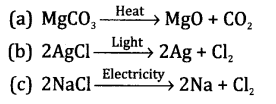Question 13.
What is the difference between displacement and double displacement reactions? Write equations for these reactions. 
In a displacement reaction, one element takes the place of another in a compound. For example,
Fe(s) + CuSO 4(aq) ➝ FeSO 4(aq) + Cu(s)
In a double displacement reaction, one com-ponent each of both the reactants get exchanged to form the products. For example,
HCl(aq) + NaOH(aq) ➝ NaCl(aq) + H 2O(l)

Question 14.
In the refining of silver, the recovery of silver from silver nitrafe solution involved displacement by copper metal. Write down the reactions involved.
The chemical equation for the displacement reaction is:Question 15.
What do you mean by precipitation reaction? Explain giving examples.
In a precipitation reaction, one of the products formed during the reaction does not dissolve in solution and gets settled on the surface of the container (beaker or tube). It is known as a precipitate.
Examples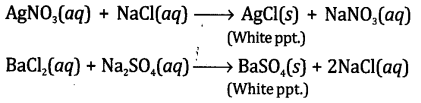Question 16.
Explain the following in terms of gain or loss of oxygen with two examples of each:
(a) oxidation
(b) reduction
(a) Oxidation involves the gain of oxygen by a substance in a chemical reaction.
Examples(b) Reduction involves the loss of oxygen from a substance in a chemical reaction.
ExamplesQuestion 17.
A shining brown-coloured element ‘X’ on heating in air becomes black in colour. Name the element ‘X’ and the black-coloured compound formed. 
The element ‘X’ is copper and the black- coloured compound formed is copper(II) oxide.Question 18.
Why do you apply paint on iron articles?
Iron is a reactive metal. In the presence of humid air (O 2 + H 2O), it rusts to form a layer of Fe 2O 3.xH 2O.
To prevent it from rusting, paint is applied which forms a protective layer over iron and prevents direct contact of air and water.

Question 19.
Oil and fat containing food items are flushed with nitrogen. Why?